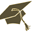Thesis (Selection of subject)(version: 290)
Assignment details
Login via CASHamiltonian and thermodynamic theory of solids and fluids
Thesis title in Czech: Hamiltonovská a termodynamická teorie pevných látek a tekutin Hamiltonian and thermodynamic theory of solids and fluids Nerovnovážná termodynamika, GENERIC, eulerovský popis Non-equilibrium thermodynamics, GENERIC, Eulerian description 2017/2018 diploma thesis angličtina Mathematical Institute of Charles University (32-MUUK) RNDr. Michal Pavelka, Ph.D. hidden- assigned and confirmed by the Study Dept. 22.01.2018 22.01.2018 01.02.2018 13.09.2019 08:00 19.07.2019 19.07.2019 Ing. Václav Klika, Ph.D. RNDr. Jaroslav Hron, Ph.D.
 Guidelines 1. Eulerian formulation of the standard reversible part of continuum mechanics, in particular with a neo-Hookean model or linearized elasticity. 2. A short introduction to the GENERIC framework. 3. Hamiltonian formulation of kinematics of deformation and comparison to the standard Eulerian equations. 4. Analytical or numerical solutions of a simple elastic problem without free boundaries formulated in the Eulerian frame. 5. Addition of dissipation into the evolution equation for the distortion matrix or left Cauchy-Green tensor and numerical solution to the simple problem with dissipation - simulation of plasticity. 6. If possible, characteristics and eigenvalues could be calculated to verify hyperbolicity of the problem. 7. If possible, free boundaries and other solution techniques (Lagrangian, ALE, Eulerian and finite elements vs. finite volumes) could be discussed.
 References  Grmela, M., A framework for elasto-plastic hydrodynamics, Physics Letters A, 2003, 312, 136  Godunov, S. K. and Peshkov, I. M, Thermodynamically Consistent Nonlinear Model of Elastoplastic Maxwell Medium, Computational Mathematics and Mathematical Physics, 2010, Vol. 50, No. 8, pp. 1409-1426  Ilya Peshkov, Michal Pavelka, Evgeniy Romenski, Miroslav Grmela, Continuum Mechanics and Thermodynamics in the Hamilton and the Godunov-type Formulations, Accepted to Continuum Mechanics and Thermodynamics (2018).
 Preliminary scope of work in English Kinematics of classical continuum mechanics is based on the notion of a reference (Lagrangian) configuration, deformation of which is studied by means of Newton laws. The reference configuration, however, loses its physical meaning for example in the case of severe plastic deformations, first-order phase transitions or chemical reactions because the mapping from the reference configuration to the current configuration no longer exists or is forgotten by the material. A way to circumvent this obstacle is to focus on fully Eulerian theories of solids and fluids by means of an underlying Hamiltonian structure. One then obtains a fully Eulerian (everything is in the current configuration) thermodynamically consistent theory of solids and fluids, which can be transformed to each other.

Charles University | Information system of Charles University | http://www.cuni.cz/UKEN-329.html